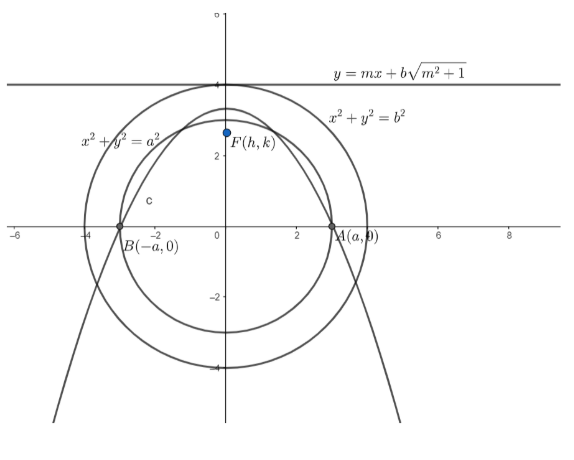Courses
Courses for Kids
Free study material
Offline Centres
MoreLast updated date: 29th Nov 2023
Total views: 383.4k
Views today: 8.83k

# A parabola is drawn to pass through $A$ and $B$, the ends of a diameter of a given circle of radius $a$ and to have as directrix a tangent to a concentric circle of radius $b$; the axes being $AB$ and a perpendicular diameter, prove that the locus of the focus of the parabola is $\dfrac{{{x}^{2}}}{{{b}^{2}}}+\dfrac{{{y}^{2}}}{{{b}^{2}}-{{a}^{2}}}=1$.Verified
383.4k+ views
Hint: Any point on the parabola is equidistant from the focus and directrix.

Let the equation of the circle be ${{x}^{2}}+{{y}^{2}}={{a}^{2}}.....\left( i \right)$
In the question, it is given that $A$ and $B$ are the ends of the diameter of the circle.So, let $A=\left( a,0 \right)$ and $B=\left( -a,0 \right)$
In the question, it is given that the radius of the concentric circle is $b$.
So, let the equation of concentric circle be ${{x}^{2}}+{{y}^{2}}={{b}^{2}}....\left( ii \right)$
Now, the directrix of the parabola is tangent to the concentric circle.
First, we find the equation of tangent to the concentric circle.
We know, the equation of tangent to a circle ${{x}^{2}}+{{y}^{2}}={{r}^{2}}$ in slope form is given as $y=mx\pm r\sqrt{1+{{m}^{2}}}$.
So, equation of tangent to $\left( ii \right)$is
$y=mx+b\sqrt{{{m}^{2}}+1}$
Or $mx-y+b\sqrt{{{m}^{2}}+1}=0....\left( iii \right)$
Equation $\left( iii \right)$ is also the directrix to the parabola.
Now, we want to find the locus of the focus of the parabola.
So, let the focus of the parabola be $F\left( h,k \right)$.
Now, by the definition of a parabola, any point on the parabola is equidistant from the focus and the directrix.
Now, from the question, we know that the ends of diameter of $\left( i \right)$i.e. $A$ and $B$ lie on the parabola.
We know that the distance between two points $({{x}_{1}},{{y}_{1}})$ and $({{x}_{2}},{{y}_{2}})$ is given as $d=\sqrt{{{({{x}_{1}}-{{x}_{2}})}^{2}}+{{({{y}_{1}}-{{y}_{2}})}^{2}}}$ and the distance of a point $({{x}_{1}},{{y}_{1}})$ from the line $lx+my+n=0$ is given
as $d=\dfrac{l{{x}_{1}}+m{{y}_{1}}+n}{\sqrt{{{l}^{2}}+{{m}^{2}}}}$.
So, for $A\left( a,0 \right)$, we have
$\sqrt{{{\left( h-a \right)}^{2}}+{{k}^{2}}}=\dfrac{m\left( a \right)-1\left( 0 \right)+b\sqrt{{{m}^{2}}+1}}{\sqrt{{{m}^{2}}+1}}$
$\Rightarrow \sqrt{{{\left( h-a \right)}^{2}}+{{k}^{2}}}=\dfrac{am+b\sqrt{{{m}^{2}}+1}}{\sqrt{{{m}^{2}}+1}}....\left( iv \right)$
For $B\left( -a,0 \right)$, we have
$\sqrt{{{\left( h+a \right)}^{2}}+{{k}^{2}}}=\dfrac{m\left( -a \right)-1\left( 0 \right)+b\sqrt{{{m}^{2}}+1}}{\sqrt{{{m}^{2}}+1}}$
$\Rightarrow \sqrt{{{\left( h+a \right)}^{2}}+{{k}^{2}}}=\dfrac{-am+b\sqrt{{{m}^{2}}+1}}{\sqrt{{{m}^{2}}+1}}....\left( v \right)$
Adding $\left( iv \right)$and $\left( v \right)$, we have
$\sqrt{{{\left( h-a \right)}^{2}}+{{k}^{2}}}+\sqrt{{{\left( h+a \right)}^{2}}+{{k}^{2}}}=\dfrac{2b\sqrt{{{m}^{2}}+1}}{\sqrt{{{m}^{2}}+1}}$
$\Rightarrow \sqrt{{{\left( h-a \right)}^{2}}+{{k}^{2}}}+\sqrt{{{\left( h+a \right)}^{2}}+{{k}^{2}}}=2b$
$\sqrt{{{\left( h+a \right)}^{2}}+{{k}^{2}}}=2b-\sqrt{{{\left( h-a \right)}^{2}}+{{k}^{2}}}$
Now, on squaring both sides we get,
${{h}^{2}}+2ah+{{a}^{2}}+{{k}^{2}}=4{{b}^{2}}+{{h}^{2}}-2ah+{{a}^{2}}+{{k}^{2}}-4b\sqrt{{{(h-a)}^{2}}+{{k}^{2}}}$
\begin{align} & \Rightarrow 4{{b}^{2}}-4ah=4b\sqrt{{{(h-a)}^{2}}+{{k}^{2}}} \\ & \Rightarrow {{b}^{2}}-ah=b\sqrt{{{(h-a)}^{2}}+{{k}^{2}}} \\ \end{align}
Now, let’s square both sides again to remove the square root sign. We get ${{b}^{4}}+{{a}^{2}}{{h}^{2}}-2{{b}^{2}}ah={{b}^{2}}({{h}^{2}}-2ah+{{a}^{2}}+{{k}^{2}})$
On dividing both sides with ${{b}^{2}}$, we get
${{b}^{2}}+\dfrac{{{a}^{2}}{{h}^{2}}}{{{b}^{2}}}-2ah={{h}^{2}}-2ah+{{a}^{2}}+{{k}^{2}}$
$\Rightarrow {{b}^{2}}-{{a}^{2}}-{{k}^{2}}={{h}^{2}}-\dfrac{{{a}^{2}}{{h}^{2}}}{{{b}^{2}}}$
$\Rightarrow ({{b}^{2}}-{{a}^{2}})(1-\dfrac{{{k}^{2}}}{{{b}^{2}}-{{a}^{2}}})={{h}^{2}}(1-\dfrac{{{a}^{2}}}{{{b}^{2}}})$
$\Rightarrow ({{b}^{2}}-{{a}^{2}})(1-\dfrac{{{k}^{2}}}{{{b}^{2}}-{{a}^{2}}})={{h}^{2}}(\dfrac{{{b}^{2}}-{{a}^{2}}}{{{b}^{2}}})$
$\Rightarrow 1-\dfrac{{{k}^{2}}}{{{b}^{2}}-{{a}^{2}}}=\dfrac{{{h}^{2}}}{{{b}^{2}}}$
$\Rightarrow 1=\dfrac{{{h}^{2}}}{{{b}^{2}}}+\dfrac{{{k}^{2}}}{{{b}^{2}}-{{a}^{2}}}$
So, the locus of $(h,k)$ is given by replacing $(h,k)$by $(x,y)$
So, the equation of the locus is given as $\dfrac{{{x}^{2}}}{{{b}^{2}}}+\dfrac{{{y}^{2}}}{{{b}^{2}}-{{a}^{2}}}=1$ 

Note: The distance between two points $({{x}_{1}},{{y}_{1}})$ and $({{x}_{2}},{{y}_{2}})$ is given as $d=\sqrt{{{({{x}_{1}}-{{x}_{2}})}^{2}}+{{({{y}_{1}}-{{y}_{2}})}^{2}}}$ and not $d=\sqrt{{{({{x}_{1}}+{{x}_{2}})}^{2}}+{{({{y}_{1}}+{{y}_{2}})}^{2}}}$. It is a very common mistake made by students.